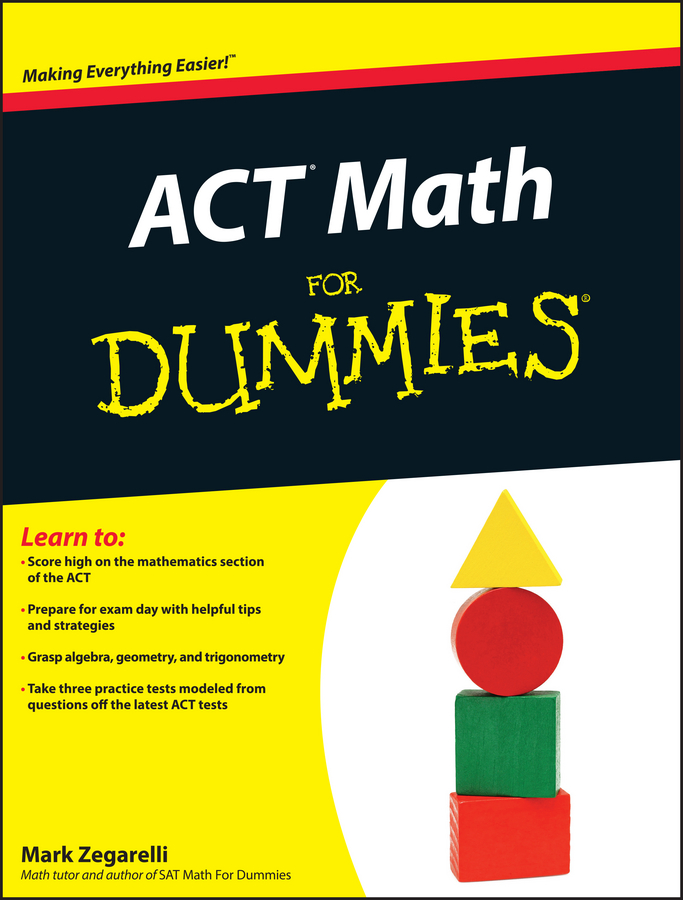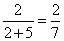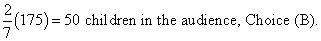##### ACT Math For DummiesOn the PSAT/NMSQT math sections, you may find questions about ratios. Percents are all about the relationship between a part and the whole. Ratios express the relationship between parts.

You hear references to ratios all the time when people say things like, “last season that pitcher delivered seven strikeouts for every nine walks,” or, “the ratio of peas to carrots in the average box is eight to one.” Notice that in these comments you aren’t given the total number of batters the pitcher faced or the amount of chopped-up veggies you may have for dinner.

Again, ratios are about parts, not wholes.

Ratios are usually written with a colon (one dot atop another), this way:

The ratio of slurps to burps is 12:5.

When you read that sentence aloud, the colon becomes “to,” as in, “the ratio of slurps to burps is 12 to 5.”

On the PSAT/NMSQT, you may be asked about possible totals. The sum of the parts is a possible total, but so are all the multiples of that sum. So if the ratio of biology majors to French majors is 4 to 3, the total number of French and biology majors may be 7, 14, 21, 28 . . . you get the idea!

If you’re asked to say what is or must be the total, the answer is cannot be determined, because any multiple of 7 is possible, based on the sum of the ratio parts.

Backsolving helps with ratios. If you’re asked about a possible total, look for an answer choice that’s a multiple of the sum of the parts.

Sometimes the test-makers give you the total and the ratio and ask you to figure out how many are in each part, as in this question:

George gobbles 2 jelly beans for every 3 gummy worms. If George has 75 pieces of candy, how many jelly beans does he have?

George will have a major stomachache, but no worries for you, because you simply follow these steps:

You know George’s sweet stash contains jelly beans and gummy worms in a 2:3 ratio, and 2 + 3 = 5.

2. Divide the total by the sum you calculated.

Okay, 75 divided by 5 gives you a quotient (what you get when you divide) of 15.

3. Multiply each part of the ratio by the quotient.

So you have 2 x 15, which equals 30 jelly beans, and 3 x 15, which equals 45 gummy worms.

Your total should be 75, and 30 + 45 does indeed equal 75.

Gobble up a gummy-whatever and turn your attention to these questions.

1. The ratio of children to adults in a movie theater is 2:5. If the theater has 175 people, how many of them are children?

(A) 2
(B) 50
(C) 100
(D) 125
(E) 150
2. A bag contains marbles and dice. If the ratio of marbles to dice in the bag is 4:5, what is one possible total number of marbles and dice in the bag?

(A) 27
(B) 28
(C) 29
(D) 30
(E) 31
3. Chester Middle School’s chess team has a winning to losing ratio of 9:4. If the team won 99 of the chess games that they played, how many games did they play altogether?

(A) 140
(B) 141
(C) 142
(D) 143
(E) 144

1. B. 50

If the ratio of children to adults is 2:5, then children make up the following portion of the audience.Now just multiply the proportion of children by the total number of people:2. A. 27

You know that for every 4 marbles there are 5 dice, so your total number of marbles and dice must be a multiple of 4 + 5 = 9. Choice (A) is the only one that’s a multiple of 9, so it’s the one you’re looking for!

3. D. 143

To get from the winning ratio to the winning number of games, you just need to multiply by 11 (because 99 = 9 x 11), so the number of games lost must also be the ratio of games lost multiplied by 11, or 44 games. Add together 99 winning and 44 losing games, and you have a total of 143 chess games. Checkmate with Choice (D)!